In: Finance

# After adding a new line of widgets, Worldwide expects all assets and current liabilities to shrink...

After adding a new line of widgets, Worldwide expects all assets and current liabilities to shrink with sales. the company has sales for the year just ended of $20 million. The company also has a profit margin of 20 percent, a return ratio of 25 percent, and expected sales of$18 million next year.

Worldwide Widgets Manufacturing, Inc., shows the following on its balance sheet:

 Assets Liabilities & Equity Current Assets $2,500,000 Current liabilites$1,250,000 Fixed Assets $3,500,000 Long-term Debt$1,500,000 Equity $3,250,000 Total Assets$6,000,000 Total Liabilities & Equity $6,000,000 What amount of additional funds (AFN) will worldwide need from external sources to fund the expected growth? What does the AFN show? Please show all detailed calculations. Also kindly put the answers not on pen and paper but typed instead. Thanks ## Solutions ##### Expert Solution Answer: Additional funds needed =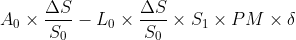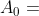Current level of assets =$ 6 million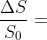Percentage increase in sales (i.e Change in sales divide by current sales)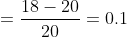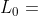Current level of liabilities (current liabilities is to shrink with sales)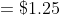million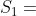new level of sales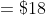millon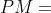Profit margin = 20%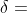retention ratio = 1-payout ratio

= 25%

Additional funds needed(AFN) = 6 * (-0.1) - 1.25 * (-0.1) * 18 * 20% * 25%

= - $0.4875million The AFN shows that external funding is not required which is in line since sales are decreasing from 20million to 18million after introducing the new line. Incase the question had been that the sales increases by 18million after introduction of this line then the AFN would be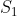= 20+18 =$38 million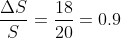AFN = 6 * 0.9 - 1.25 * 0.9 * 38 * 20% * 25%